# Recursive Sequences In Math(Edited)

Hi there. In this mathematics post I cover recursive number sequences in mathematics. A recursive sequence is a mathematical pattern that refers to a previous number as its input. If that definition sounds confusing I do have examples later in this post.

The screenshots are from using witeboard.com and my small Wacom Tablet with Stylus Pen.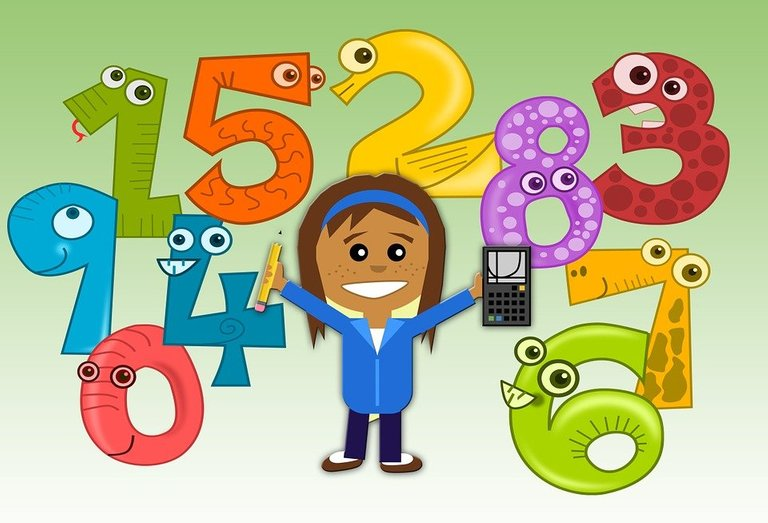Pixabay Image Source

## Topics

• Number Patterns As Sequences
• Arithmetic Sequences
• Geometric Sequences
• Writing A Sequence As Recursion
• Using A Recursive Formula
• Extension - A Look At The Fibonacci Sequence Of Numbers

## Number Patterns As Sequences

In numbers, life and in nature we see & notice patterns in a variety of places. We see patterns in nature, numbers, clothing, food, financial markets, art and more. Here we deal with number patterns such as:

2, 4, 6, 8, 10, 12

1, 0, 1, 0, 1, 0, ...

1, -3, 9, -27, +81, -243, ...

Note that the last number pattern is an alternating sequence. It is alternating in the sense that the sign changes from positive to negative and vice versa. In fact, you multiply by negative 3 to get the next number.

## Arithmetic Sequences

When a number pattern involve adding or subtracting by a constant amount that number pattern is an arithmetic sequence. The positive even numbers is an arithmetic sequence as it starts from 2 and adds by 2 each time to get the next number. That is `2, 4, 6, 8, 10, 12, 14, 16, 18, ...`.

Multiples of 10 is another arithmetic sequence as it is `10, 20, 30, 40, 50, ...`. Add 10 to get the next number.

An arithmetic sequence that involves subtraction would be `100, 95, 90, 85, 80, ... , 0`. This is starting at 100 with subtracting 5 each time to get the next number.

## Geometric Sequences

Geometric sequences involve multiplication and division to obtain the next number in the number pattern.

Starting from 10, you can multiply by 10 each time to obtain the geometric sequence of `10, 100, 1000, 10 000, 100 000, 1 million, 10 million, ...`.

There is also the case where you divide by a number. This geometric sequence starts at 16 and we divide by 2 to get the next number. Note that dividing by 2 is the same as taking half of a number.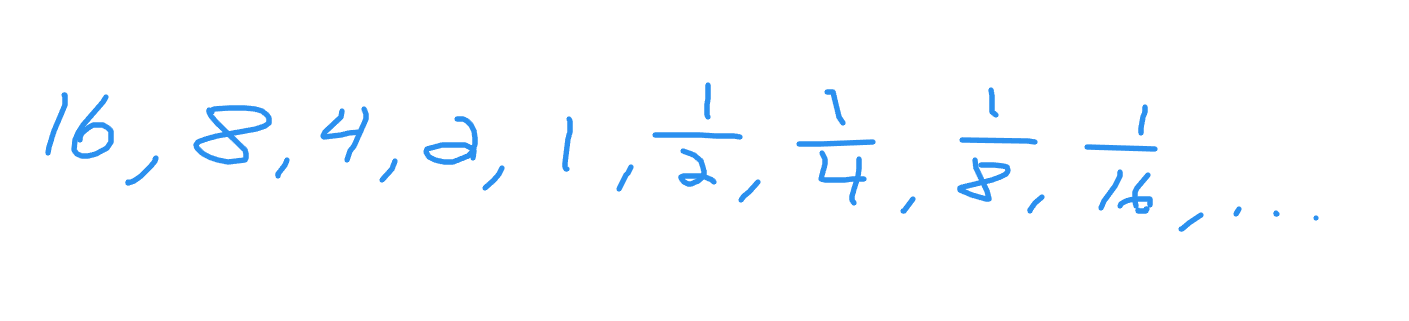## Writing A Sequence As Recursion

So far I have provided the basics of number sequences/patterns. These number sequences can be written in such a way that we use the previous number to get the next number in a compact mathematical form.

Example One - Arithmetic Sequence

Suppose you have the arithmetic sequence of `7, 14, 21, 28, 35` and so on. These numbers are the multiples of 7 or the seven times tables numbers.

The first number or first term is 7. To get the next number just add by 7. Define `n` as a positive whole number starting at 1. Also let the first term be `t_1 = 7`. The second term would be the first term plus 7. This would be 14. The third number would be 14 + 7.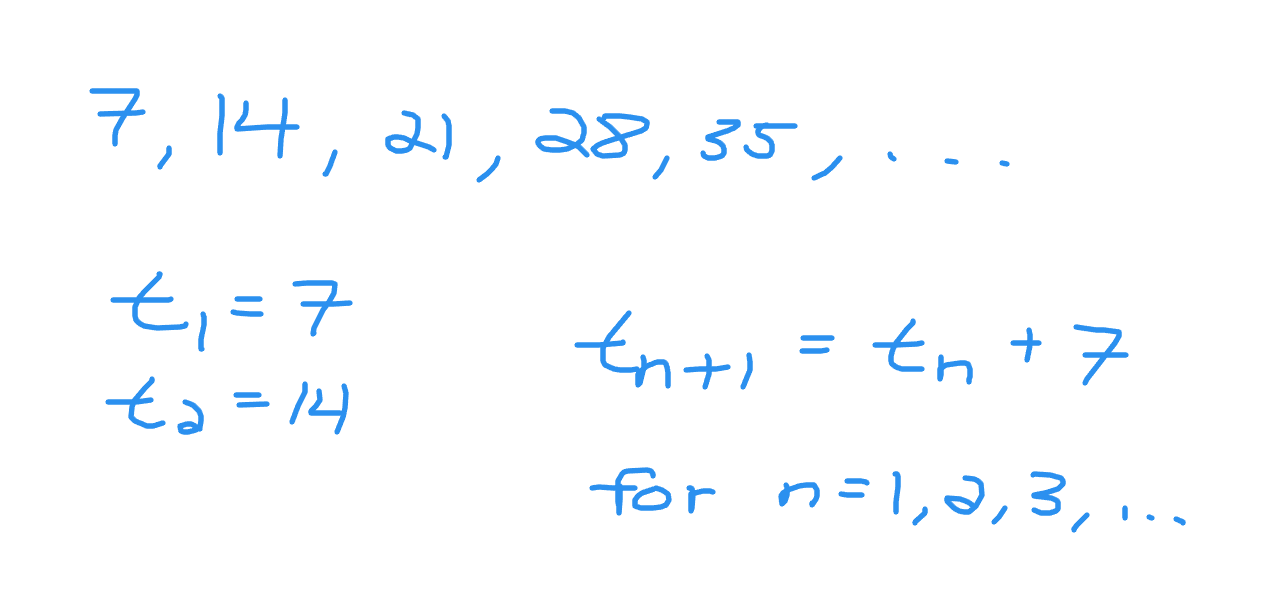Example Two - Geometric Sequence

What would the recursive equation be for the geometric sequence of `2, 4, 8, 16, 32, 64` and so on?

The first term is 2. Multiplying by 2 from 2 gives `2 x 2 = 4` as the second term. The general rule is to multiply by 2 to get the next number.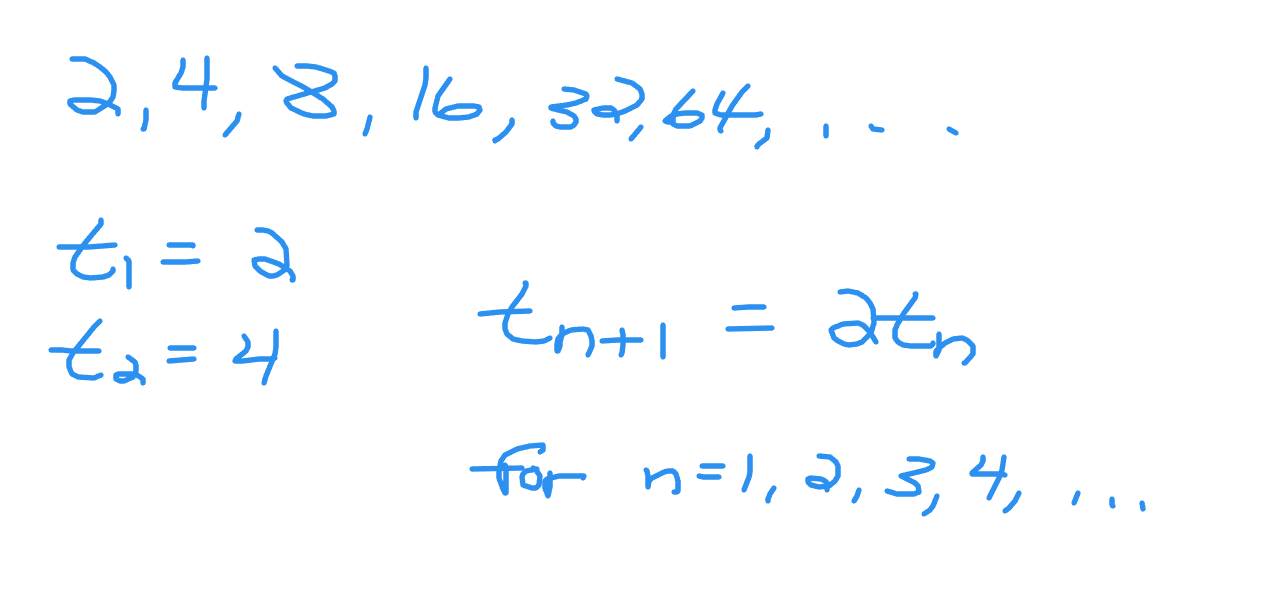Example Three - Alternating Sequence

You have this alternating sequence of `-1, +1, -1, +1`. Notice that the sign changes from positive to negative and vice versa when it comes to obtaining the next term number. What is the recursive formula for this case?

One may look at this as +2 and minus 2 for the pattern rule but this is not consistent. If you look at this more like a geometric sequence you will see that we multiply by negative one. The one is the same number but the sign changes.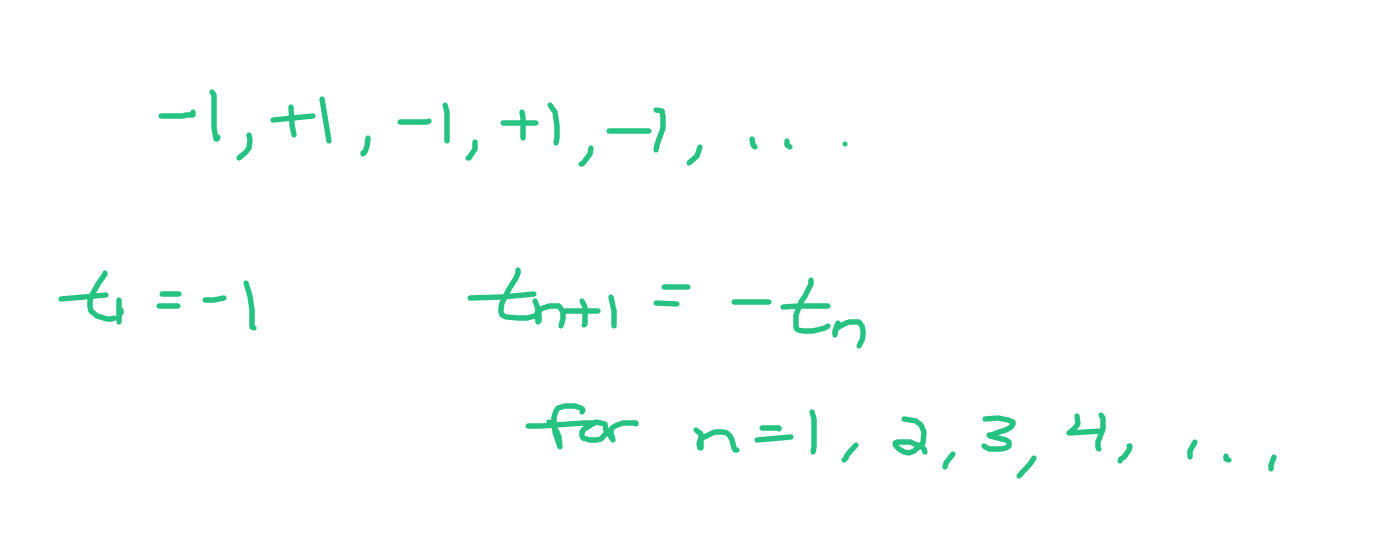## Using A Recursive Formula

The previous section was about going from the number pattern to writing it down algebraically in recursive form. In this section, the recursive formula is given and numbers are then obtained from the formula to generate a sequence of numbers.

In each of these three example, the first five terms are determined given the recursive formula.

Example One - Arithmetic Sequence Formula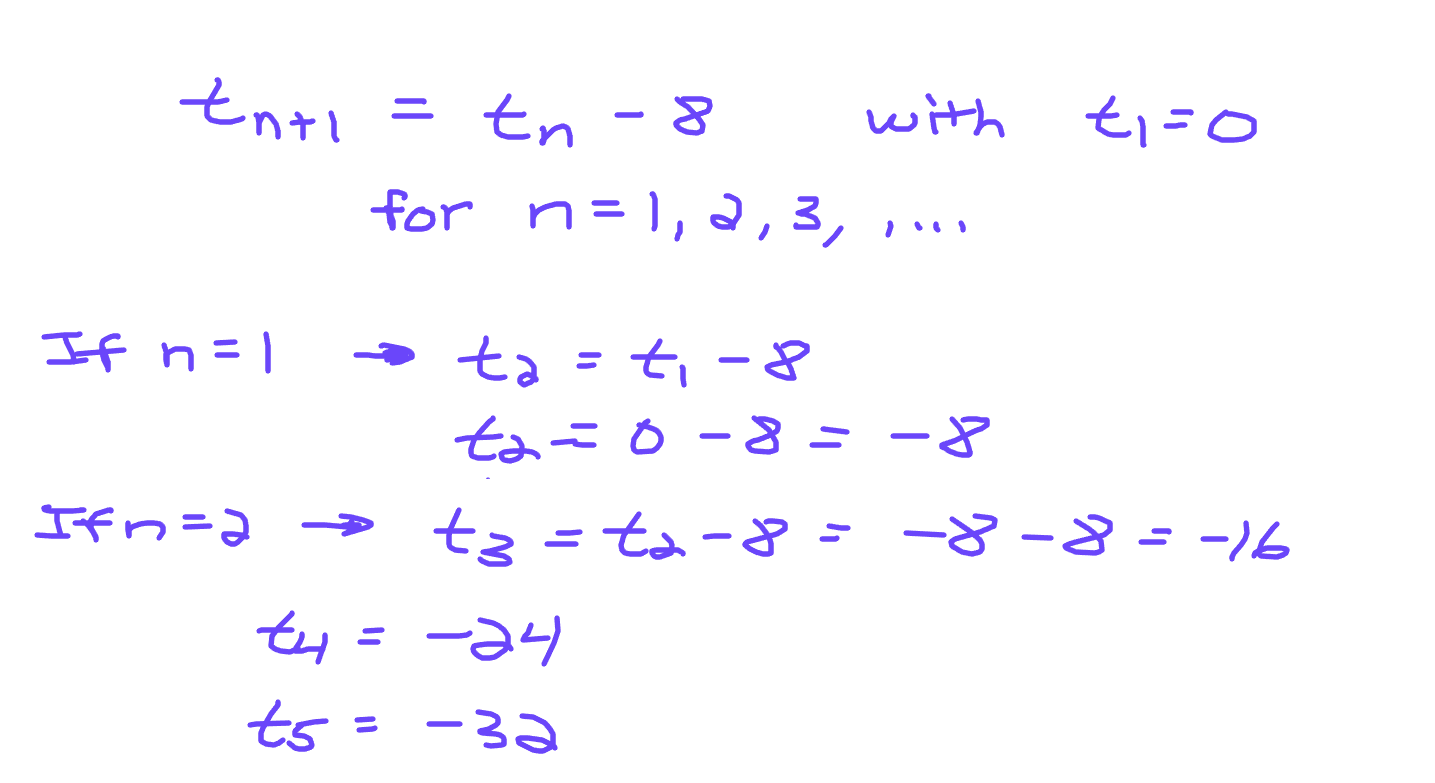Example Two - Geometric Sequence Formula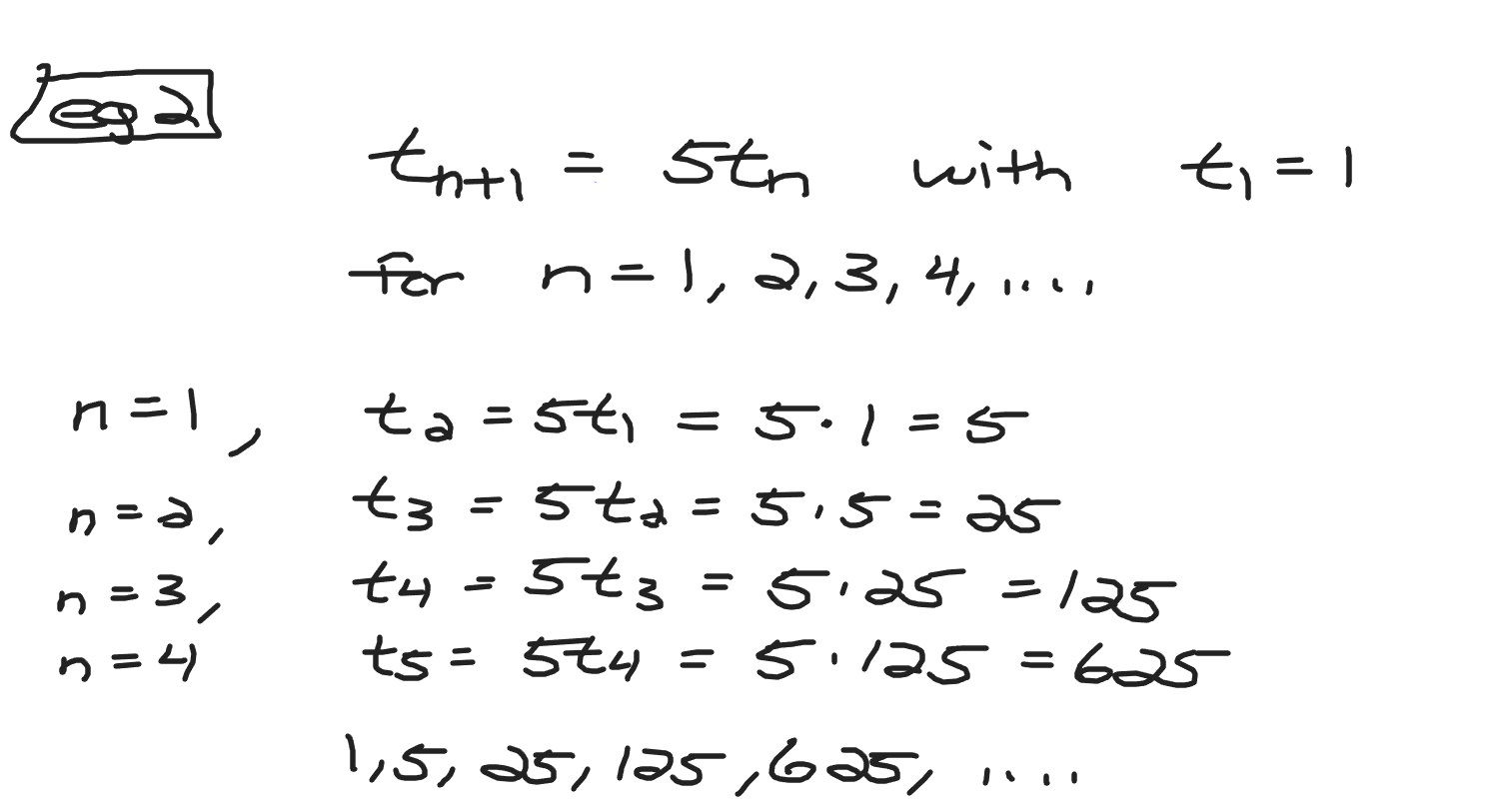Example Three - Alternating Sequence Formula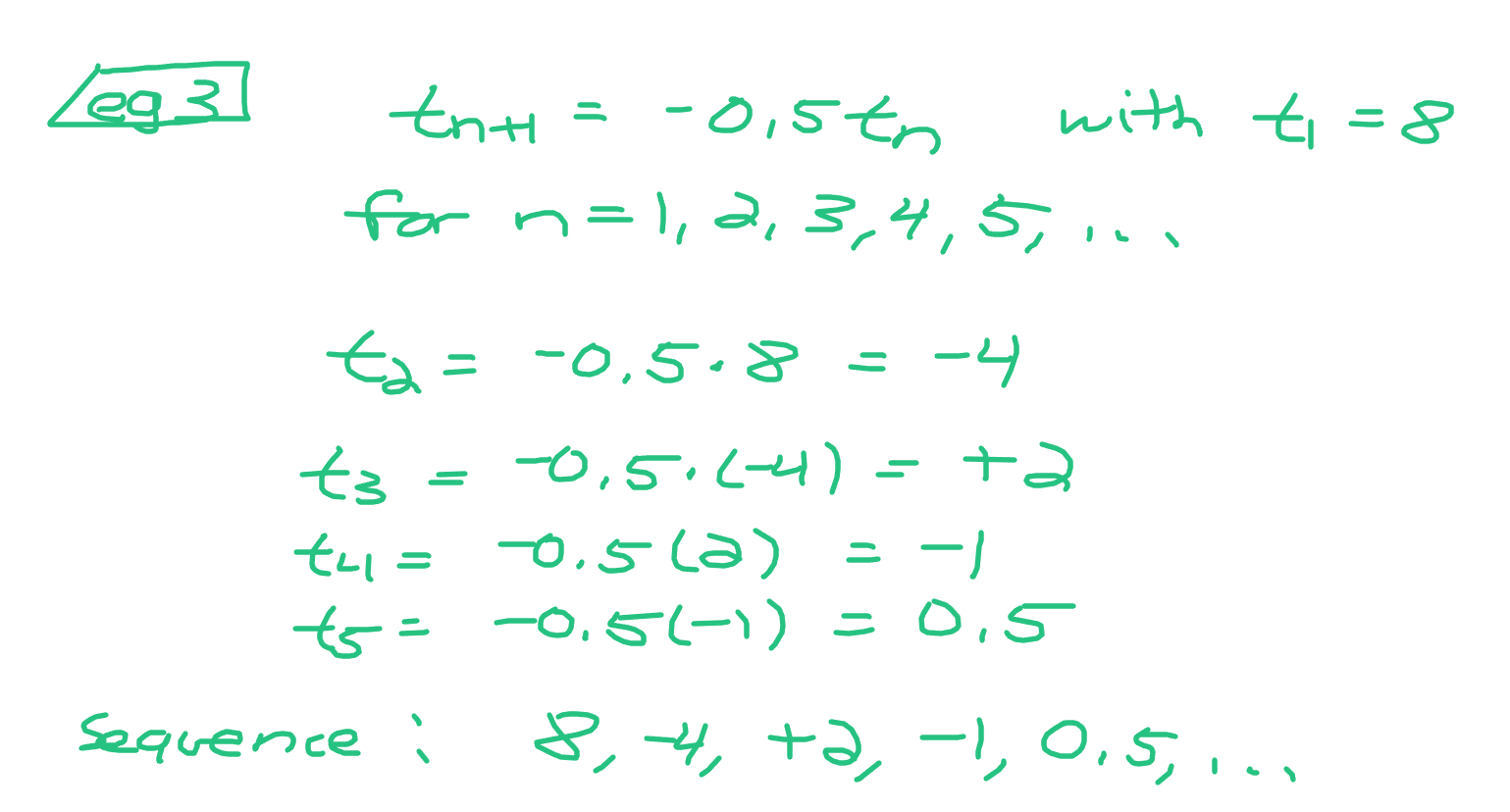## Extension - A Look At The Fibonacci Sequence Of Numbers

The Fibonacci sequence of numbers is an interesting case. It is a number pattern that is recursive in nature. Let's take a look at the Fibonacci numbers first.

1, 1, 2, 3, 5, 8, 13, 21, 34, ...

This Fibonacci sequence starts with 1 and 1 as the first two terms. To obtain the two as the third term add the two previous numbers together. The three is from adding the two previous numbers being 1 and 2. In general, the next number is obtained by adding the two previous numbers.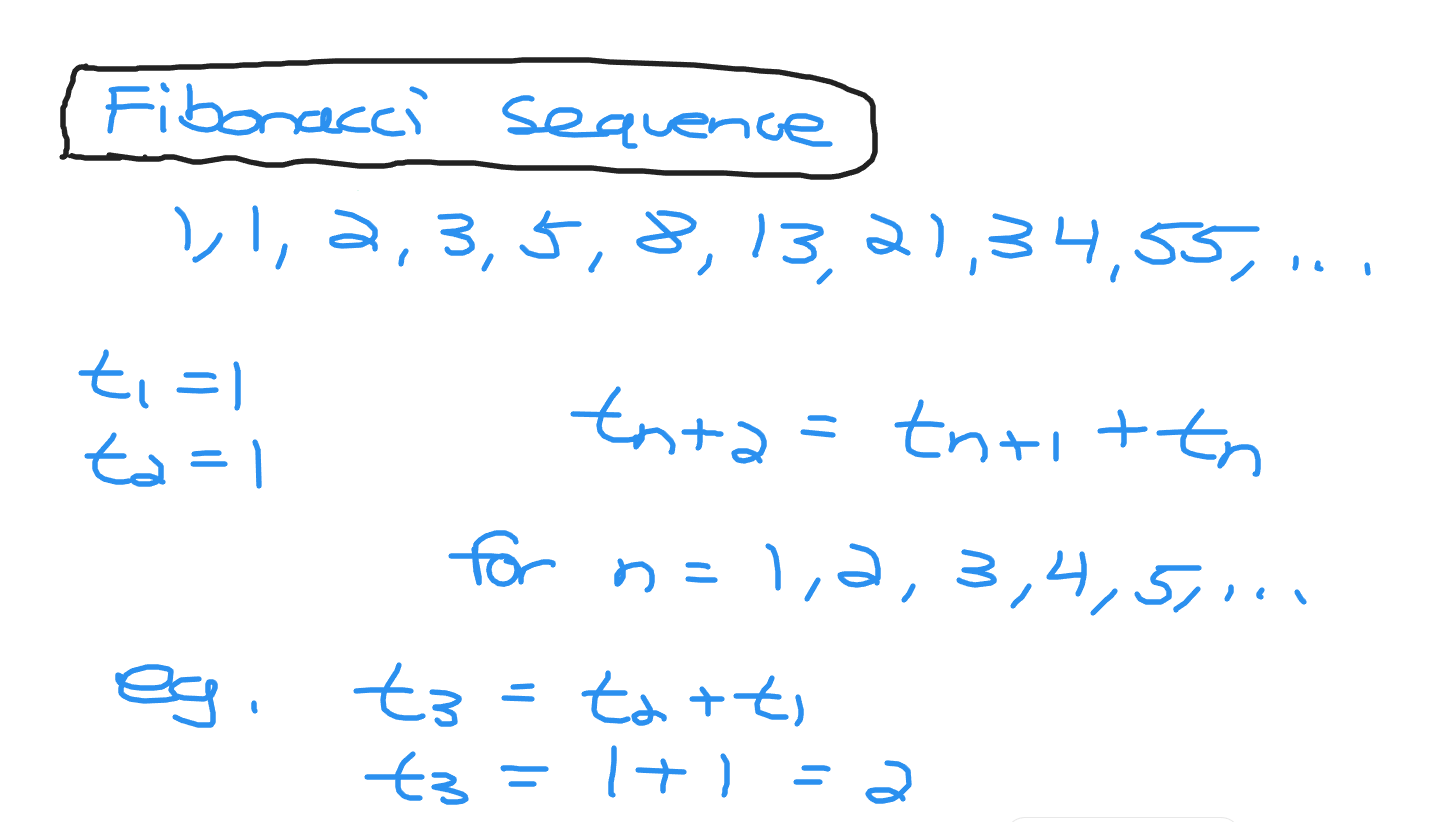Posted with STEMGeeks

0
0
0.0000
0
0.000#### You have received a 1UP from @gwajnberg!

The @oneup-cartel will soon upvote you with:
@stem-curator
And they will bring !PIZZA 🍕.

0
0
0.000🍕 PIZZA !

@curation-cartel(2/20) tipped @dkmathstats (x1)

Send \$PIZZA tips in Discord via tip.cc!

0
0
0.000Thanks for your contribution to the STEMsocial community. Feel free to join us on discord to get to know the rest of us!

Please consider delegating to the @stemsocial account (85% of the curation rewards are returned).

You may also include @stemsocial as a beneficiary of the rewards of this post to get a stronger support.

0
0
0.000